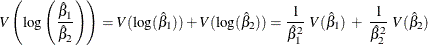### Direct Standardization

Direct standardization uses the weights from a reference population to compute the standardized rate of a study group as the weighted average of stratum-specific rates in the study population. The standardized rate is computed as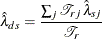where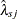is the rate in the jth stratum of the study population,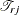is the population-time in the jth stratum of the reference population, and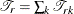is the population-time in the reference population.

Similarly, direct standardization uses the weights from a reference population to compute the standardized risk of a study group as the weighted average of stratum-specific risks in the study population. The standardized risk is computed as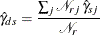where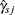is the risk in the jth stratum of the study population,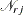is the number of observations in the jth stratum of the reference population, and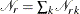is the total number of observations in the reference population.

That is, the directly standardized rate and risk of a study population are weighted averages of the stratum-specific rates and risks, respectively, where the weights are the corresponding strata population sizes in the reference population. The direct standardization can be used when the study population is large enough to provide stable stratum-specific rates or risks. When the same reference population is used for multiple study populations, directly standardized rates and risks provide valid comparisons between study populations.

The variances of the directly standardized rate and risk are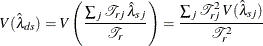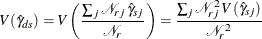By using the method of statistical differentials (Elandt-Johnson and Johnson, 1980, pp. 70–71), the variance of the logarithm of directly standardized rate and risk can be estimated by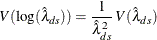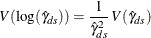The confidence intervals for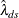and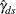can be constructed based on normal and lognormal distributions. A gamma distribution confidence interval can also be constructed for.

In the next four subsections,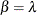denotes the rate statistic and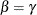denotes the risk statistic.

#### Normal Distribution Confidence Intervals for Standardized Rate and Risk

A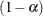confidence interval for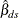based on a normal distribution is then given by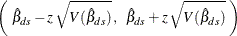where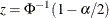is the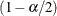quantile of the standard normal distribution.

#### Lognormal Distribution Confidence Intervals for Standardized Rate and Risk

Aconfidence interval for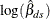based on a normal distribution is given by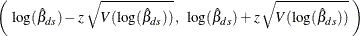whereis thequantile of the standard normal distribution.

Thus, aconfidence interval forbased on a lognormal distribution is given by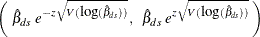#### Gamma Distribution Confidence Interval for Standardized Rate

Fay and Feuer (1997) use the relationship between the Poisson and gamma distributions to derive approximate confidence intervals for the standardized ratebased on the gamma distribution. As in the construction of the asymptotic normal confidence intervals, it is assumed that the number of events has a Poisson distribution, and the standardized rate is a weighted sum of independent Poisson random variables. A confidence interval foris then given by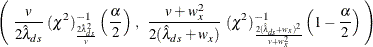where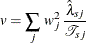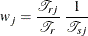and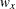is the maximum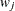.

Tiwari, Clegg, and Zou (2006) propose a less conservative confidence interval forwith a different upper confidence limit,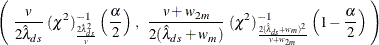where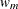is the averageand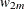is the average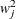.

#### Comparing Standardized Rates and Comparing Standardized Risks

By using the same reference population, two directly standardized rates or risks from different populations can be compared. Both the difference and ratio statistics can be used in the comparison. Assume that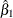and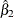are directly standardized rates or risks for two populations with variances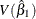and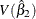, respectively. The difference test assumes that the difference statistic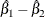has a normal distribution with mean 0 under the null hypothesis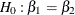. The variance is given by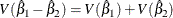The ratio test assumes that the log ratio statistic,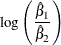has a normal distribution with mean 0 under the null hypothesis, or equivalently,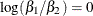. An estimated variance is given by